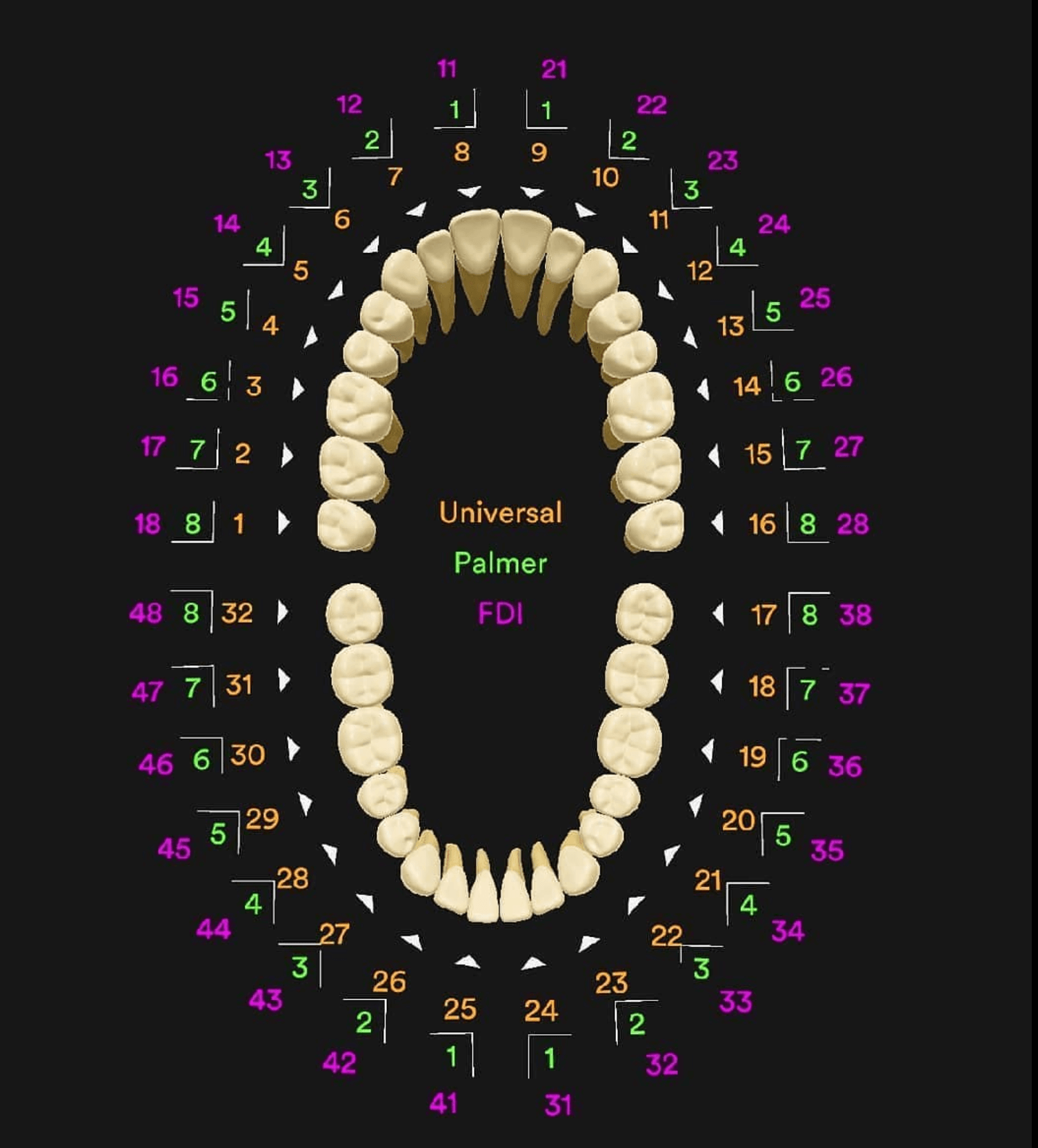• 30 November, 2022

#### Tooth Numbering Systems

Human beings are diphyodonts i.e., we have two sets of teeth- 20 primary and 32 permanents. Therefore, it becomes important to have a proper tooth numbering system for precise identification. We mainly have three such systems, which are applicable to both primary and permanent dentition-

1. Universal system
2. Zsigmondy/ palmar system
3. FDI System

Universal system

The primary teeth in the system are denoted using alphabets written in capital letters. The numbering starts from right maxillary second molar A, and is continued sequentially across the arts up to the left maxillary second molar J. The numbering for the mandibular arch begins with the letter K, denoting the left mandibular second molar and is continued sequentially up to the right mandibular second molar T.

Similarly, the permanent teeth under the system are denoted using numbers from 1 to 32. It again starts from the right side from maxillary third molar being denoted by number 1 and continues across the arch with left maxillary third molar denoted by number 16. The numbering is continued with left mandibular third molar which is denoted as 17 and is continued to number 32 denoting the right mandibular third molar.

Zsigmondy/ Palmer System

This system is represented using a horizontal and a vertical line. The horizontal line divides the maxillary and mandibular arch where are the vertical line represents the mid sagittal plane. Each quadrant is assigned a quadrant bracket that surrounds the alphabet or number denoting the tooth.

The primary teeth are numbered with capital letters from A to E with A being the central incisor and E representing the second molar. These letters are written adjacent to the quadrant bracket which represents the quadrant in which the tooth is present.

Similarly, the permanent teeth are numbered from 1 to 8 in all four quadrants with 1 being the central incisor and 8, the third molar. These numbers are again written along with the quadrant brackets that denote the exact location of the tooth in the oral cavity.

FDI System

This is a two-digit system, where the first digit indicates the quadrant and the second digit indicates the tooth within that quadrant.

For the primary teeth the quadrants are numbered from 5 to 8 in the clockwise manner with maxillary right quadrant given the number 5 and mandibular right quadrant is number 8. The second digit is number from 1 to 5 representing the five teeth in the particular quadrant from Central incisor to second molar respectively.

For example, the number 63 denotes a deciduous canine present in the maxillary left quadrant.

For the permanent teeth the quadrants are numbers from 1 to 4 in the same clockwise fashion. The teeth present in a particular quadrant and number from 1 to 8 with 1 being The Central incisor and 8 denotes the third molar. For example, the number 37 denotes the second molar present in mandibular left quadrant.

Note- FDI system has been adopted by WHO and International Association for Dental Research, and is the universally accepted system of tooth numbering.